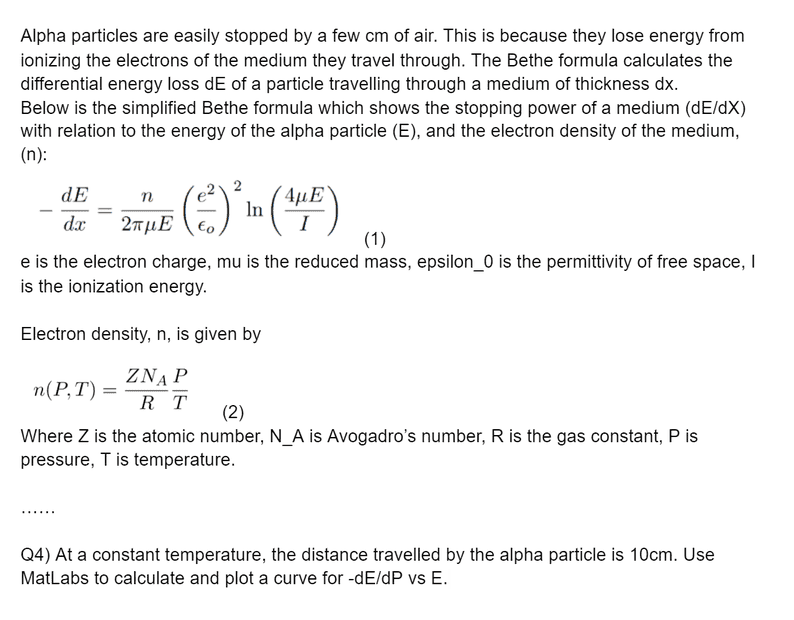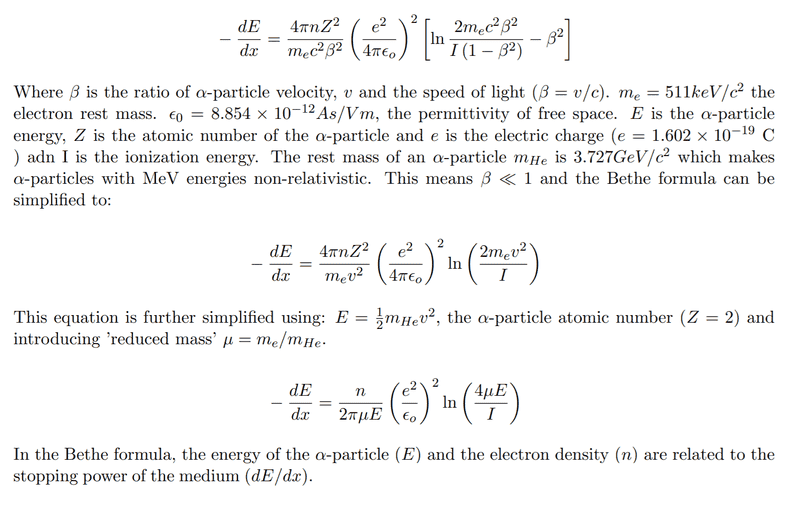# Estimating the energy of an alpha particle using Bethe's formula

PandaKitten
Homework Statement:
Full question is below
Relevant Equations:
Bethe's formula
The question is below. I tried reasoning that because x is constant, E is also constant however that gives me values in the range of 10^51. Then I tried to use numpy's ivp_solve function to solve the differential equation however I wasn't able to get that working either. Apparently I'm meant to use Taylor's expansion to estimate E however I'm not sure how I would do that.Homework Helper
Gold Member
This is a simplified version which we were given because the alpha particle is non relativistic so beta << 1 and it also uses reduced mass mu and also E = 1/2 m_e/m_He.
This last statement is unclear. Please show what ## \mu ## is algebraically. Supposedly ## E ## is the energy of the alpha particle. This looks like a case where your notes that you might have taken in lecture contain errors.

PandaKitten
This is what the question statesHomework Helper
Gold Member
I have to admit, I can't make sense out of their asking for a graph of ## -\frac{dE}{dP} ## vs. ## E ##. Perhaps someone else can see what they are asking for here.

kdv
Homework Statement:: Full question is below
Relevant Equations:: Bethe's formula

The question is below. I tried reasoning that because x is constant, E is also constant however that gives me values in the range of 10^51. Then I tried to use numpy's ivp_solve function to solve the differential equation however I wasn't able to get that working either. Apparently I'm meant to use Taylor's expansion to estimate E however I'm not sure how I would do that.
View attachment 282789
Are you 100% sure they are asking a graph of -dE/dP vs E?? I would be willing to bet that this is a typo and they really are just asking a plot of -dE/dx vs E, which is then simple, they just want the graph of the expression they provided. (By the way, their definition of reduced mass is very unorthodox!)

•hutchphd
Homework Helper
2022 Award
I have to admit, I can't make sense out of their asking for a graph of −dEdP vs. E. Perhaps someone else can see what they are asking for he
For instance, one might wish to choose a pressure where the response of the detector has particular characteristics for a certain energy alpha particle. Maybe there is a nice flat spot or an edge.

Staff Emeritus